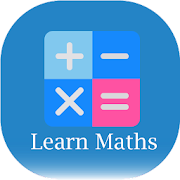# Math Quiz, Learn Add,Subtraction,Multiply & Divide APK    
5/5
5 Ratings
Developer
Current Version
1.2
Date Published
File Size
11M
Package ID
Price
\$ 0.00
100+
Category
Android Apps
Genre
Education

## APK Version History

Version
1.2 (*)
Architecture
all
Release Date
August 02, 2020
Requirement
Android 5.0 and up
Safe
•••••This is a Math learning app for all ages of peoples to learn math addition, subtraction, multiplication & division with the help of this math quiz / math game app.
This app is step wise first easy then slowly start hard parts
Math Quiz, Learn Add,Subtraction,Multiply & Divide - Maths Tricks app is free for everyone all ages of people. To learn Mathematical calculations & practice to learn maths addition subtraction multiplication & division. The math quiz / math game app division calculator is for all ages of people to learn mathematics easily by using this mathematics solution app as the maths division app & the math division game also called them math divide app math division app. This is best math quiz brain maths game as exercise math free maths games very useful and helpful and many stages of this maths game & maths quiz app this math games for peoples to train brain . The math game for adults also the addition games as the math test plus minus is very useful mathematics apps.

Features of Math Quiz, learn add, Subtraction, multiply, divide - Maths Tricks
- (Subtraction)
* (Multiplication)
/ (Division)
^ (Exponent: "raised to the power")
√ (Square Root)
|x| (Absolute Value of x